A wire of length L and 3 identical cells of negligible internal resistances are connected in series. Due to current, the temperature of the wire is raised by ΔT in a time t. A number N of similar cells is now connected in series with a wire of the same material and cross–section but of length 2 L. The temperature of the wire is raised by the same amount ΔT in the same time t. the value of N is

(1) 4

(2) 6

(3) 8

(4) 9

Concept Questions :-

Grouping of cells
High Yielding Test Series + Question Bank - NEET 2020

Difficulty Level:

What is the equivalent resistance between the points A and B of the network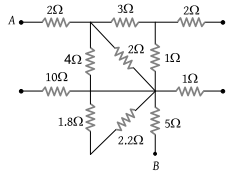(1) $\frac{57}{7}\Omega$

(2) 8 Ω

(3) 6 Ω

(4) $\frac{57}{5}\Omega$

Concept Questions :-

Combination of resistors
High Yielding Test Series + Question Bank - NEET 2020

Difficulty Level:

The effective resistance between points P and Q of the electrical circuit shown in the figure is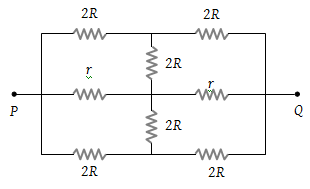(1) $2Rr/\left(R+r\right)$

(2) $8R\text{\hspace{0.17em}}\left(R+r\right)/\left(3R+r\right)$

(3) $2r+4R$

(4) $5R/2\text{\hspace{0.17em}\hspace{0.17em}}+\text{\hspace{0.17em}}2r$

Concept Questions :-

Combination of resistors
High Yielding Test Series + Question Bank - NEET 2020

Difficulty Level:

In the circuit element given here, if the potential at point B, VB = 0, then the potentials of A and D are given as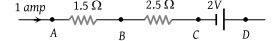(1) ${V}_{A}=-1.5\text{\hspace{0.17em}}V,\text{\hspace{0.17em}}{V}_{D}=+2\text{\hspace{0.17em}}V$

(2) ${V}_{A}=+1.5\text{\hspace{0.17em}}V,\text{\hspace{0.17em}}{V}_{D}=+2\text{\hspace{0.17em}}V$

(3) ${V}_{A}=+1.5\text{\hspace{0.17em}}V,\text{\hspace{0.17em}}{V}_{D}=+0.5\text{\hspace{0.17em}}V$

(4) ${V}_{A}=+1.5\text{\hspace{0.17em}}V,\text{\hspace{0.17em}}{V}_{D}=-0.5\text{\hspace{0.17em}}V$

Concept Questions :-

Kirchoff's voltage law
High Yielding Test Series + Question Bank - NEET 2020

Difficulty Level:

The current in a conductor varies with time t as $I=2t+3{t}^{2}$ where I is in ampere and t in seconds. The electric charge flowing through a section of the conductor during t = 2 sec to t = 3 sec is :

(1) 10 C

(2) 24 C

(3) 33 C

(4) 44 C

Concept Questions :-

Current and current density
High Yielding Test Series + Question Bank - NEET 2020

Difficulty Level:

A group of N cells whose emf varies directly with the internal resistance as per the equation EN = 1.5 rN are connected as shown in the figure below. The current I in the circuit is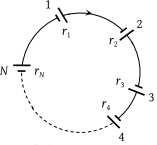1. 0.51 A

2. 5.1 A

3. 0.15 A

4. 1.5 A

Concept Questions :-

Grouping of cells
High Yielding Test Series + Question Bank - NEET 2020

Difficulty Level:

In the shown arrangement of the experiment of the meter bridge if AC corresponding to null deflection of galvanometer is x, what would be its value if the radius of the wire AB is doubled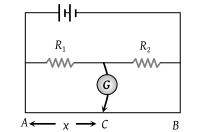(1) x

(2) x/4

(3) 4x

(4) 2x

Concept Questions :-

Meter bridge and potentiometer
High Yielding Test Series + Question Bank - NEET 2020

Difficulty Level:

Seven resistances are connected as shown in the figure. The equivalent resistance between A and B is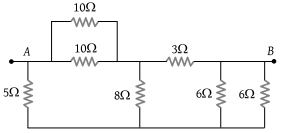(1) 3 Ω

(2) 4 Ω

(3) 4.5 Ω

(4) 5 Ω

Concept Questions :-

Combination of resistors
High Yielding Test Series + Question Bank - NEET 2020

Difficulty Level:

A battery of internal resistance 4Ω is connected to the network of resistances as shown. In order to give the maximum power to the network, the value of R (in Ω) should be :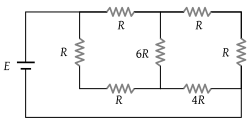(1) 4/9

(2) 8/9

(3) 2

(4) 18

Concept Questions :-

Combination of resistors
High Yielding Test Series + Question Bank - NEET 2020

Difficulty Level:

In the circuit shown here, the readings of the ammeter and voltmeter are [Kerala PMT 2002]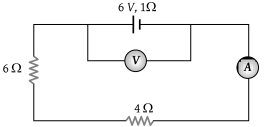(1) 6 A, 60 V

(2) 0.6 A, 6 V

(3) 6/11 A, 60/11 V

(4) 11/6 A, 11/60 V

Concept Questions :-

Kirchoff's voltage law